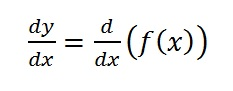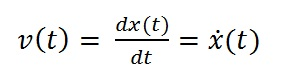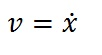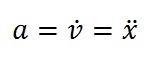# PRACTICE SET #1

(and some loose ends)

Before we get to the practice questions…

1.  I want to introduce anther notation for derivatives.

Suppose we are working with a function y=f(x)

Instead of writing the derivative as y’ we often write:The expression is telling you to find the derivative of the function f with respect to the variable x.  For now, this seems like a more cumbersome notation but there will be times when you appreciate its clarity.  It leaves no doubt about what variable you are working with.  This will be critical when you are working with functions of more than one variable.

2. And yet another notation for derivatives, this time a lazier one…

In many instances, the functions we are considering will be functions of time, t.  When we want to indicate that we are taking the derivative with respect to time, we just write a dot over the variable.  So for example, let x(t) be the position of an object as a function of time.  In that case, the velocity would be:Typically, if we are being this terse, we may as well be briefer and write:Here we are figuring that by using the dot notation, we are implicitly saying that these are both functions of time.

And then we can say things like:This is just a concise short-hand for “acceleration is the derivative of velocity with respect to time and the second derivative of position with respect to time.”

OK, this brings us to the practice set.  I have posted it here as a pdf.  Note to my Holmdel students:  I have also emailed it to your school account.  So if you did not get it, you should email me to ask to be added to the mailing list.  If you have any questions, post them here as comments (Holmdel student or not).

Practice Set #1 AP Physics Math Preview